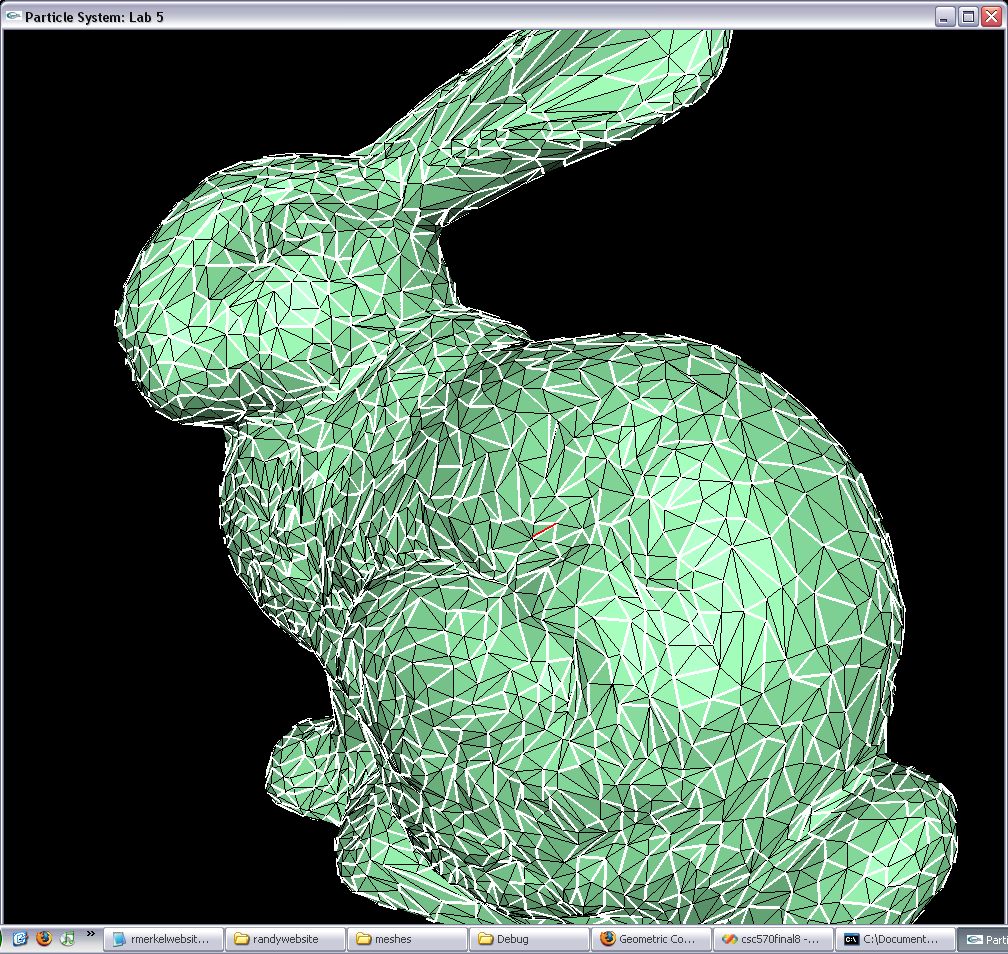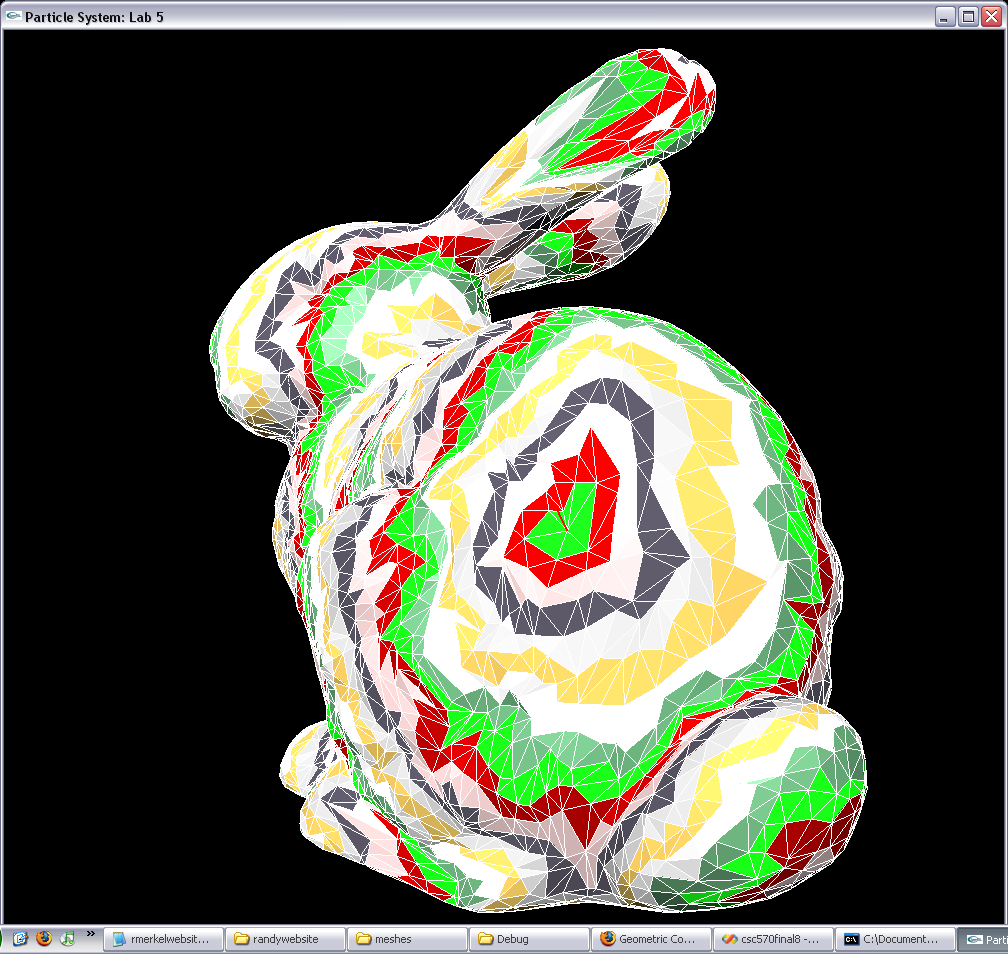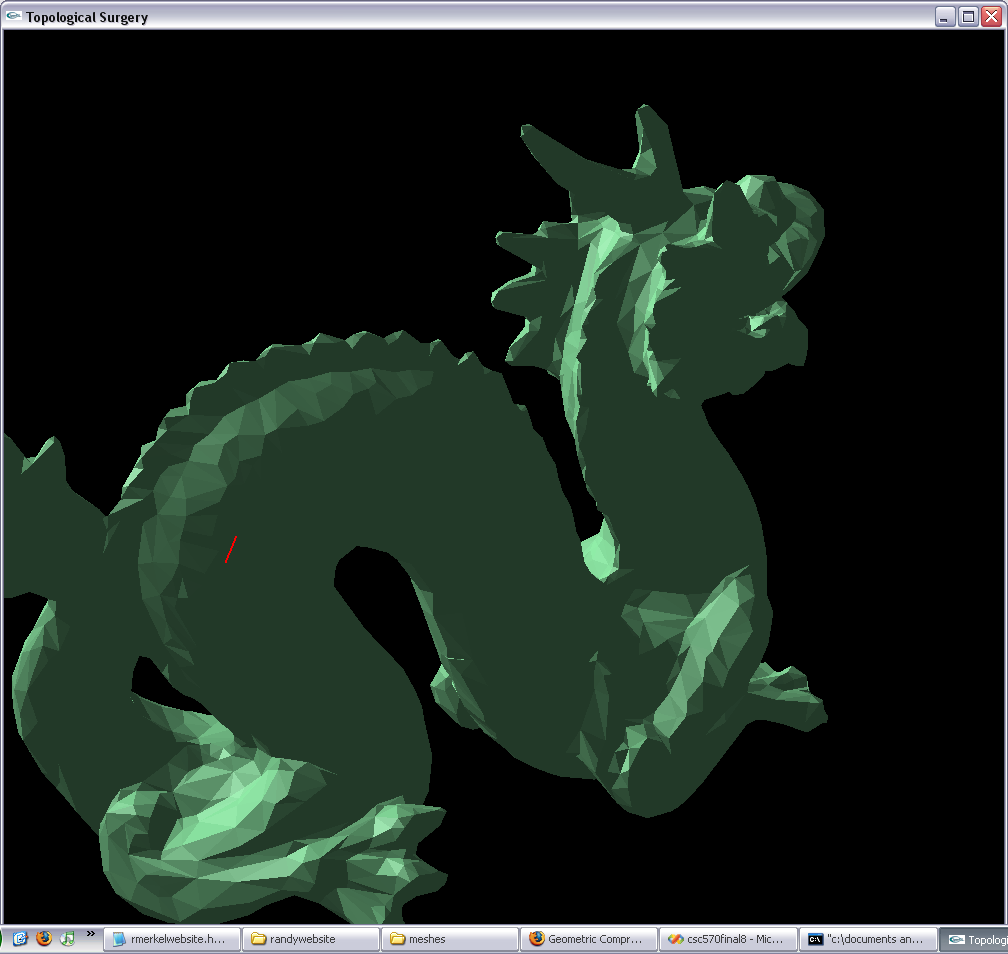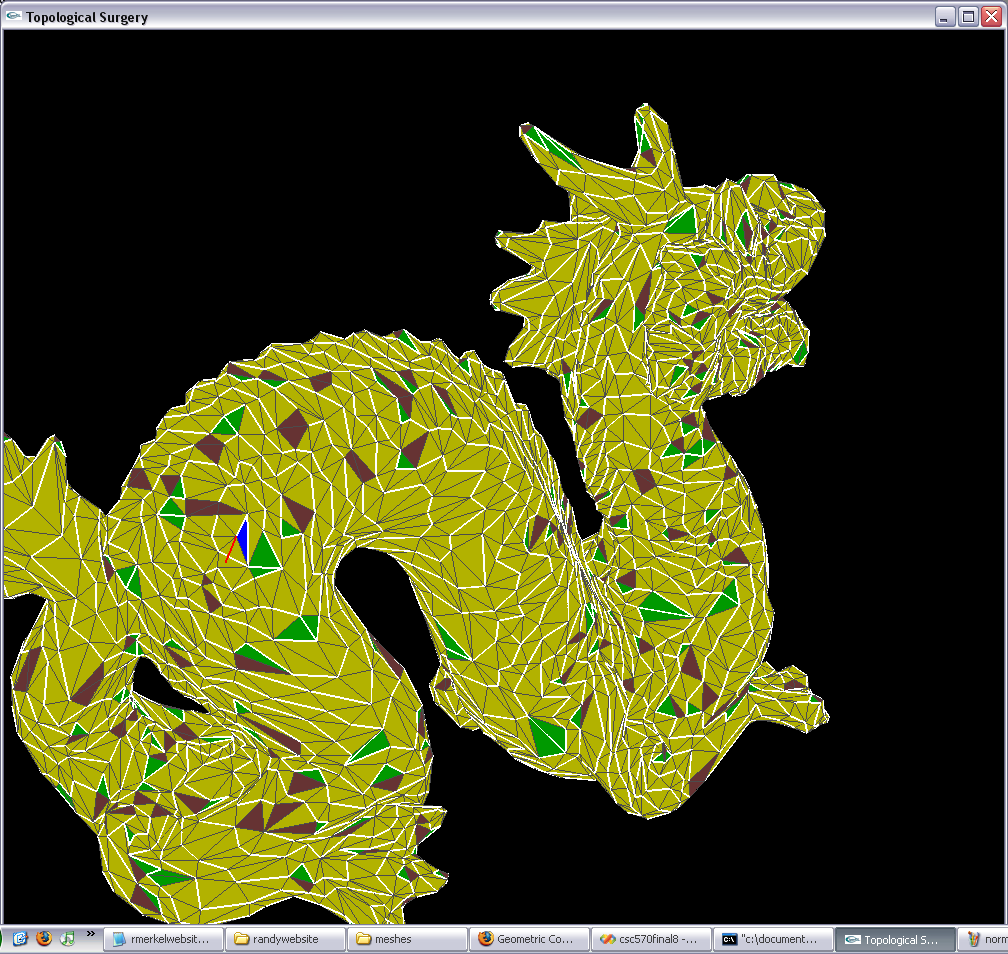Introduction

I have worked on implementing Taubin et al. paper Geometric Compression through Topological Surgery. The goal of the paper is to create a compression scheme to efficiently store and transfer complex 3D meshes.

The basic approach is to take an arbitrary manifold mesh and partition it into triangle strips. Triangle strips are an efficient way to represent connectivity information between triangle faces in a mesh. Standard triangulated meshes contain redundancy in that each face references three vertices and thus their common edges reference the same vertex multiple times.

Triangle strips operate by defining a base triangle then building off of that triangle by only keeping track of a bit to indicate which of two possible edges to build off of and the index of the next vertex to add. Each triangle strip has a small amount of overhead so the goal of any partitioning scheme is to create long triangle strips.

Their approach to partitioning a mesh into triangle strips is to create a vertex spanning tree and a triangle spanning tree for the mesh. The vertex spanning defines the borders of the triangle strips and the triangle tree defines the strips themselves and the connections between the strips. The ends of triangles trips are known as leaf nodes and the triangles that connect triangle strips are known as branching nodes.

They presented two primary ways to construct the two spanning trees:

1. 1. Pick a root vertex. Sort the edges of the mesh according to the distance from their midpoints to the root vertex. Pick the edge with the minimum distance and put that in the vertex spanning tree if it does not create a cycle in the tree. Repeat this until every vertex in the mesh is connected via this tree.
2. 2. Pick a root vertex and make it the first boundary. Find all of the faces incident on that vertex and make that the first layer. Find all of the edges in the first layer that are not incident on the first boundary and make them the second boundary. Find all of the triangles incident on the second boundary and not in the first layer, this becomes the second layer. Continue this process until the entire mesh has been covered. Merge the layers by adding edges connecting the boundaries and removing any cycles from the boundaries.
The first method produces reasonable results but the second method is much better.

The paper outlines many more objetives to compress a triangulated mesh such as vertex position prediction, entropy encoding position corrections, and compact representations of the vertex spanning tree, triangle spanning tree, and boundary formed by cutting the mesh along the vertex tree. My primary focus was on generating the triangle strips.

Screenshots

<
ScreenshotsThis is an image of the vertex spanning tree created by the first approach described above. The red line indicates the root vertex.This is an image of the first stage of the second approach before the rings have been merged.This is an image of the original mesh that is partitioned below.This is an image of the full partitioned mesh using the boundaries and layers after merging the layers. The blue face is the root of the triangle tree, the brown faces are branching nodes, and the green faces are leaf nodes.This is an image of the same partitioning as above but the problem faces are highlighted in red. The red indicates faces that are visited more than once during the tree traversal which removes back edges from the graph.

<
Results

Unfortunately the problem shown in figure 4 shows that there was somehow a loop in the triangle tree generated by my project. I was unable to find the reason for this and ran several tests and confirmations (as shown in figure 5) but none of them found the problem. Since there was a loop, I was unable to finish implementing their entire algorithm.

I could have taken what I had and made triangle strips out of them, stored those strips and compared the file size to the initial mesh file but I had the goal of finishing the entire project rather than testing the effeciency as I progrogressed. I intend to minimally find out why a loop is created and see where my compression ratio lies after that.

Here is the source for my project in .zip format: final project

References

G. Taubin and J. Rossignac,  "Geometric Compression through Topological Surgery," ACM Transactions on Graphics, April 1998.   (pdf format)